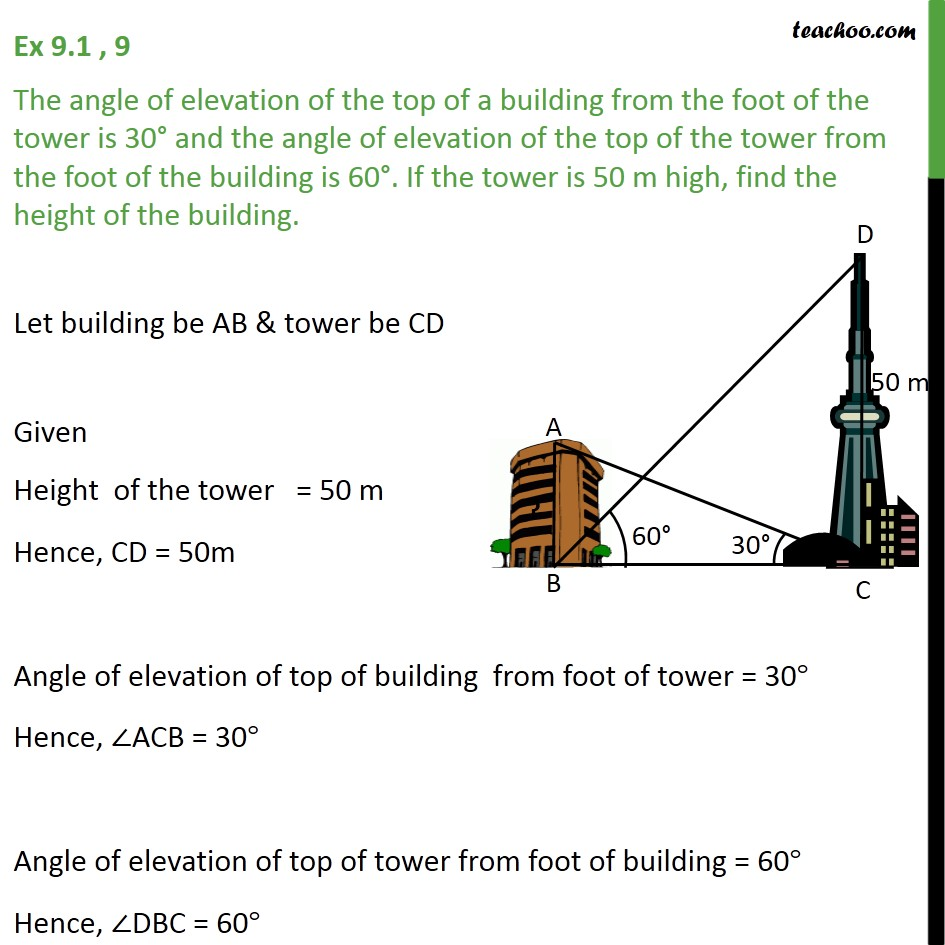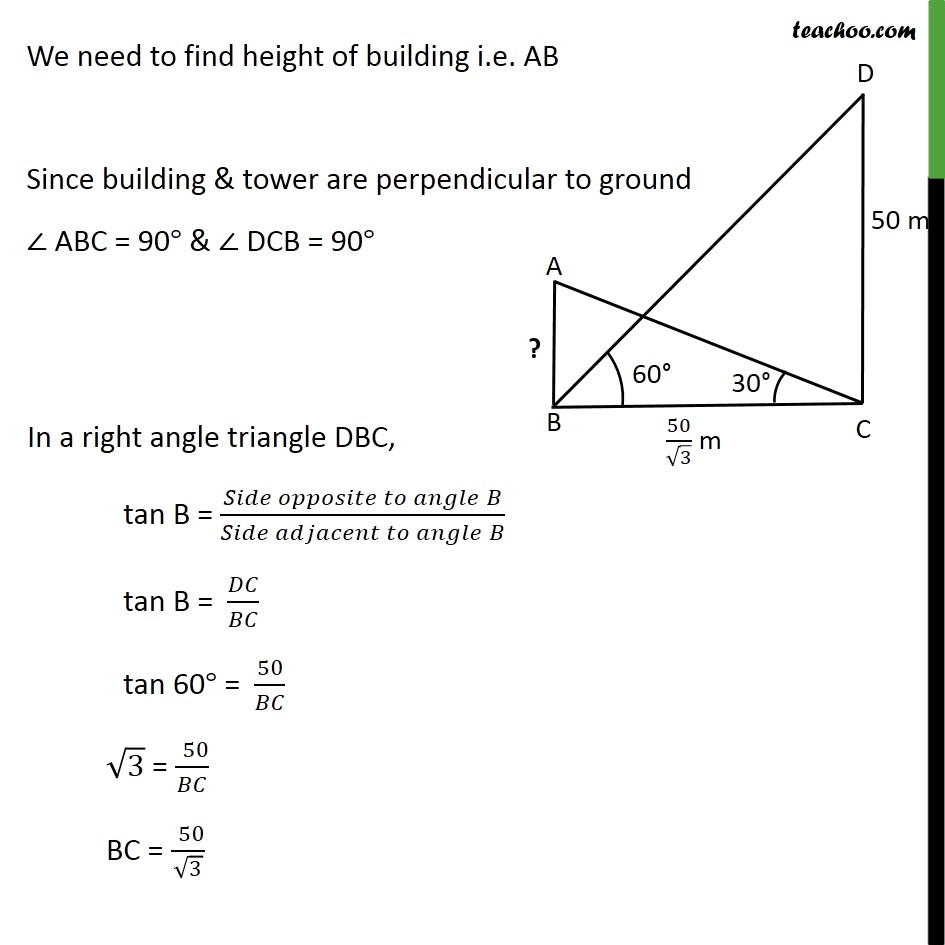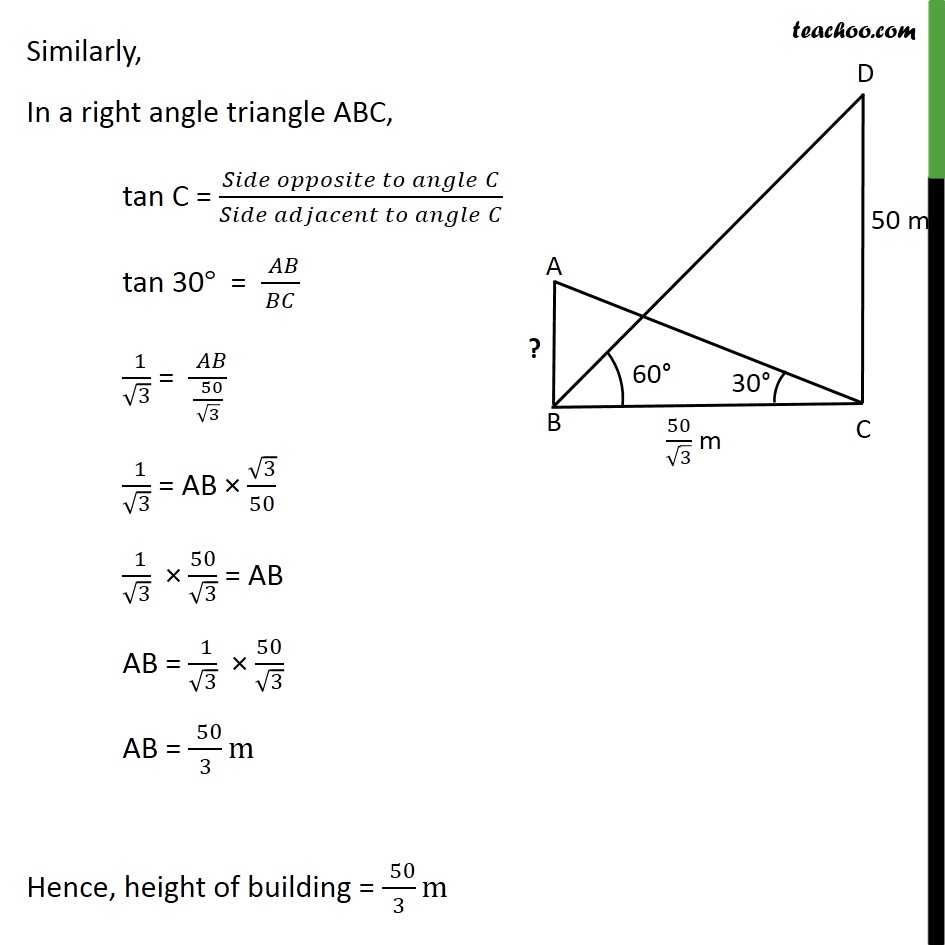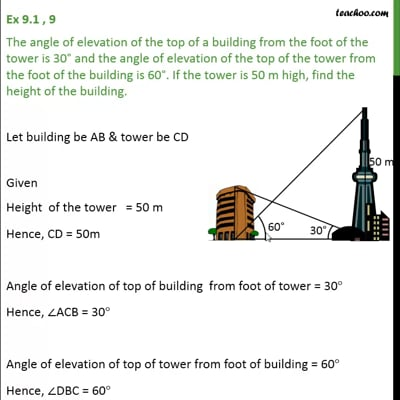Ex 9.1

Chapter 9 Class 10 Some Applications of Trigonometry
Serial order wiseThis video is only available for Teachoo black users

Solve all your doubts with Teachoo Black (new monthly pack available now!)

### Transcript

Ex 9.1 , 9 The angle of elevation of the top of a building from the foot of the tower is 30 and the angle of elevation of the top of the tower from the foot of the building is 60 . If the tower is 50 m high, find the height of the building. Let building be AB & tower be CD Given Height of the tower = 50 m Hence, CD = 50m Angle of elevation of top of building from foot of tower = 30 Hence, ACB = 30 Angle of elevation of top of tower from foot of building = 60 Hence, DBC = 60 We need to find height of building i.e. AB Since building & tower are perpendicular to ground ABC = 90 & DCB = 90 Similarly, In a right angle triangle ABC, tan C = ( " " )/( " " ) tan 30 = (" " )/ (" " 1)/ 3 = (" " )/((" " 50)/ 3) (" " 1)/ 3 = AB 3/50 (" " 1)/ 3 50/ 3 = AB AB = (" " 1)/ 3 50/ 3 AB = (" " 50)/3 m Hence, height of building = (" " 50)/3 m